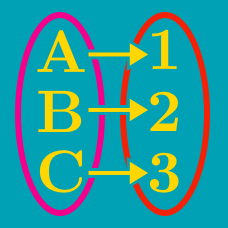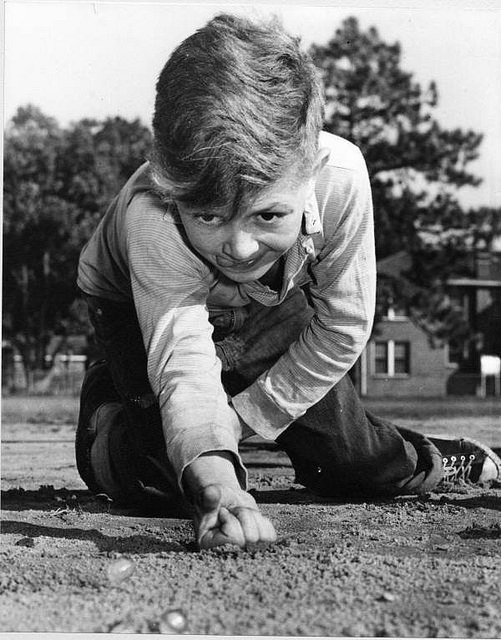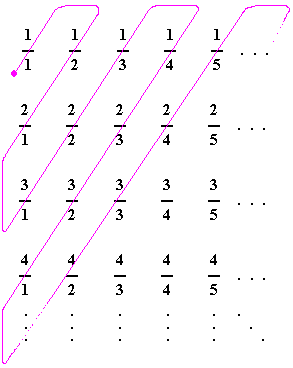Probability

# Bijections: Level 3 ChallengesAlice and Bob are at either end of a (really long) track, whose width is the diameter of a marble. Alice has 30 red marbles and Bob has 20 blue marbles. They send all of their marbles towards each other in quick succession. Whenever 2 marbles collide on the track, they will just bounce back and start traveling in the opposite direction.

When all is said and done, how many red marbles does Bob end up with?

Assume that

• All marbles move at the same speed.
• All the marbles are of the same mass,
• All the collisions are perfectly elastic, meaning that they will bounce back at the same speed.
• There isn't space for the marbles to "pass over" each other. They will collide on the track.Which of the following statements is/are true?

$A)$ The set of all real numbers in the interval $(0,1)$ is not countable.

$B)$ The set of all rational numbers in the interval $[0,1]$ is not countable.

The number of triangles with integer sides and perimeter $n$ is equal to :

Note: In the options, distinct means "pairwise distinct".

In the World Series, 2 baseball teams play till one of the teams wins exactly 4 games. How many ways are there for a (given) team to win the world series? For example, WLWWW and WWLLWW are valid orders.

Details and assumptions

LLWWLL is not considered a valid order; we want exactly 4 W's. WWLLWW is considered a valid order for the other team, and we do not want to double count.

Consider the set $\{ 1, 2, 3, 4, 5, 6, 7, 8, 9, 10 \}$. For each subset, calculate the sum of the elements in the subset. How many distinct sums can we get?

Details and assumptions

The empty subset $\{ \}$ is a valid subset.

The empty sum (sum of no elements) is 0.

×